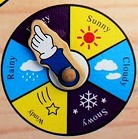# Lesson Plan of Time and Date

Lesson Plan of Time and Date with Reference to Activities

Subject Mathematics

Students` Learning Outcomes

• Recognize the hour and minute hands of an analog clock.
• Read and tell time in hours from the analog clock e.g. two o` clock.

Information for Teachers

• Students understand the concept of time with reference to activities they perform, such as time to eat, time to sleep, time to bath, time to go and time to play. Relate the time with respect to time of day: as; morning, afternoon, and night, etc.
• You can tell what time it is in several ways: as;
1. Position of the sun in the sky
• The activities people are doing and clock and watch.

Material / Resources

Writing board, chalk/marker, duster, model of clock, paper plate, print of clock with number and without number, clock, paper strip, gum, worksheet, ruler, pencils

Introduction

 Activity 1 Ask the students: as; What time do you get up in the morning? What time do you come to school? At what time do you go home? How do we know what time it is? What do you use to tell the time?

Development

 Activity 1 Show the students a clock and ask them what it is?What do we use this for? How many numbers can you see on the clock face? How many hands does the clock have? Are they the same length? Ask them if they know what the 2 hands show? Tell them that the longer hand is called the minute hand and the shorter is called the hour hand. Show different times on the clock and guide the students to read the time. Repeat each time ‘if the hour hand is on 10 and the minute hand is on 12 its 10 o` clock.

 Activity 1 Ask students to draw a number line on a strip of paper which contains the numerals 1 to 12. Show the number line on the writing board. Instruct them to bend the number line into a circle to resemble a clock face.Activity 3 Write time from 1 O`clock to 12 O`clock on separate cards and numbers from 1 to 12 on square cards. Place the numbers 1 to 12 in a large circle to form a clock face. Make students sit around the clock. Give 12 students each a time card to keep face down. Ask two volunteers of different heights to stand in the center of the clock. Ask from students: as; If these 2 are the hands of the clock then who should be the minute hand? Why? (the taller student because minute hand is    long) Where should the taller student point to show? 1 O` clock (at 12) Where should the short student point? (At 1). Students take turns holding up their time cards. Students tell where the children representing the hands should point to show that time. Ask different pairs to come to the center and show the time. Repeat the activity until all students have had a turn to show time.

 Activity 4 Divide the class into 5 groups and provide each group with a model or a worksheet of clock.Ask them to draw the hands of the clock for the time written on the writing board (e.g. 3 O` clock, 5 O` clock, etc.)

Sum up / Conclusion

• Summarize the lesson for the students: as;
• There are 1 to 12 numerals and 2 hands on the clock one big hand called the minute hand and one small hand called the hour hand.
• Show different times on the clock and ask them to tell the time.
• Ask from students which hand is at 10 and which hand is at 12.

To show time in hours the minute hand should point to 12 and the hour hand to the digit that shows time (e.g.  To show 2 O` clock minute hand should point towards 12 and hour should point towards 2.

Assessment

• Provide a work sheet with a circle drawn on it.
•

• Write 1 to 12 numerals on them and
• Draw hour hand and minute hand
• Provide students with a worksheet that has a clock face on it with numerals. Ask them to draw the hands of the clock as they should appear according to the time indicated in the problem.
• Teacher is also required to involve the students in solving the problems given in the exercise at the end of unit/chapter.